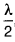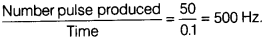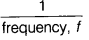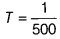# Find out wavelength, frequency and time period of the wave

A source of wave produces 50 crests and 50 trough in 0.1 s. The distance between a crest and next trough is 20 cm. Find out

1. wavelength
2. frequency and
3. time period of the wave.
1. Since, we know that the distance between a crest and next trough iswhere λ is wavelength of the wave. Therefore,= 20 cm.
∴ λ = 40 cm
2. The pluse produces by the source is 0.1 s.
∵ Frequency of a wave =3. ∵ Time period of a wave, T == 2 x 10–3s